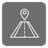Nearest Value
Elementary+
RU English JA

Find the nearest value to the given one.

You are given a list of values as set form and a value for which you need to find the nearest one.

For example, we have the following set of numbers: 4, 7, 10, 11, 12, 17, and we need to find the nearest value to the number 9. If we sort this set in the ascending order, then to the left of number 9 will be number 7 and to the right - will be number 10. But 10 is closer than 7, which means that the correct answer is 10.

A few clarifications:

• If 2 numbers are at the same distance, you need to choose the smallest one;
• The set of numbers is always non-empty, i.e. the size is >=1;
• The given value can be in this set, which means that it’s the answer;
• The set can contain both positive and negative numbers, but they are always integers;
• The set isn’t sorted and consists of unique numbers.

Input: Two arguments. A list of values in the set form. The sought value is an int.

Output: Int.

Example:

```nearest_value({4, 7, 10, 11, 12, 17}, 9) == 10
nearest_value({4, 7, 10, 11, 12, 17}, 8) == 7
```